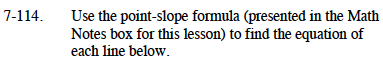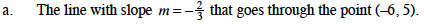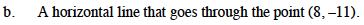### Home > CAAC > Chapter 7 > Lesson 7.3.4 > Problem7-114

7-114.Substitute the slope and the points into the point slope formula.

yk = m(xh)

$y-5 = -\frac{2}{3}(x + 6)$

$y-5 = -\frac{2}{3}x-4$

$y = -\frac{2}{3}x + 1$Substitute the slope (0 for a horizontal line) and the points into the point slope formula.

y + 11 = 0(x − 8)
yk = m(xh)
y + 11 = 0
y = −11See parts (a) and (b) to solve. Remember that perpendicular lines have opposite reciprocal slopes.